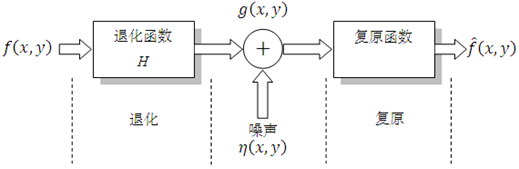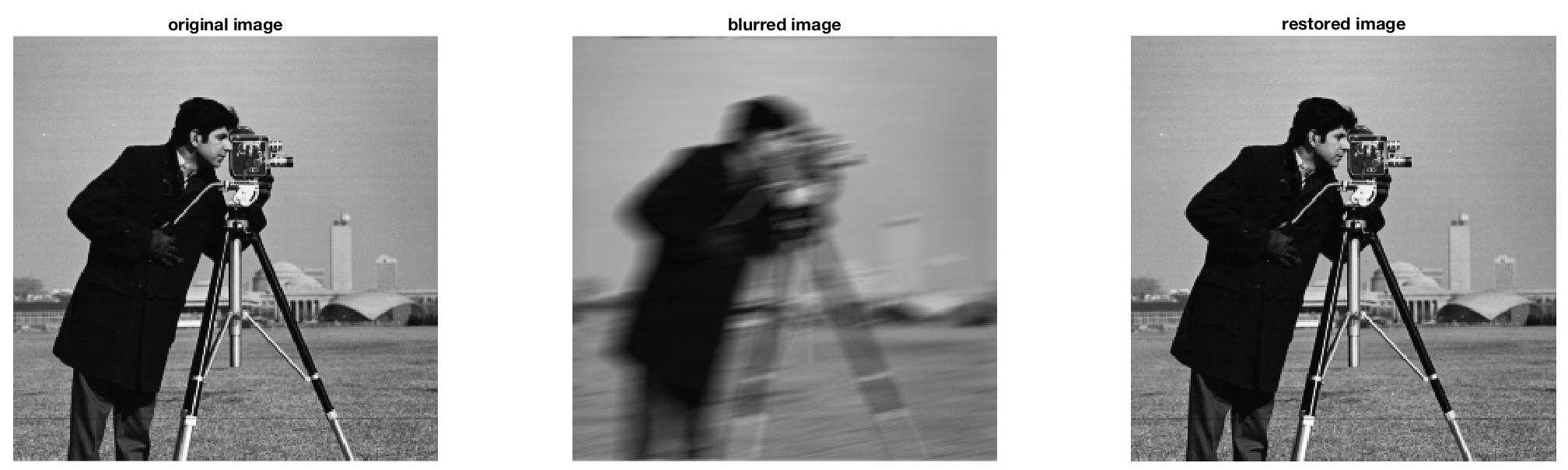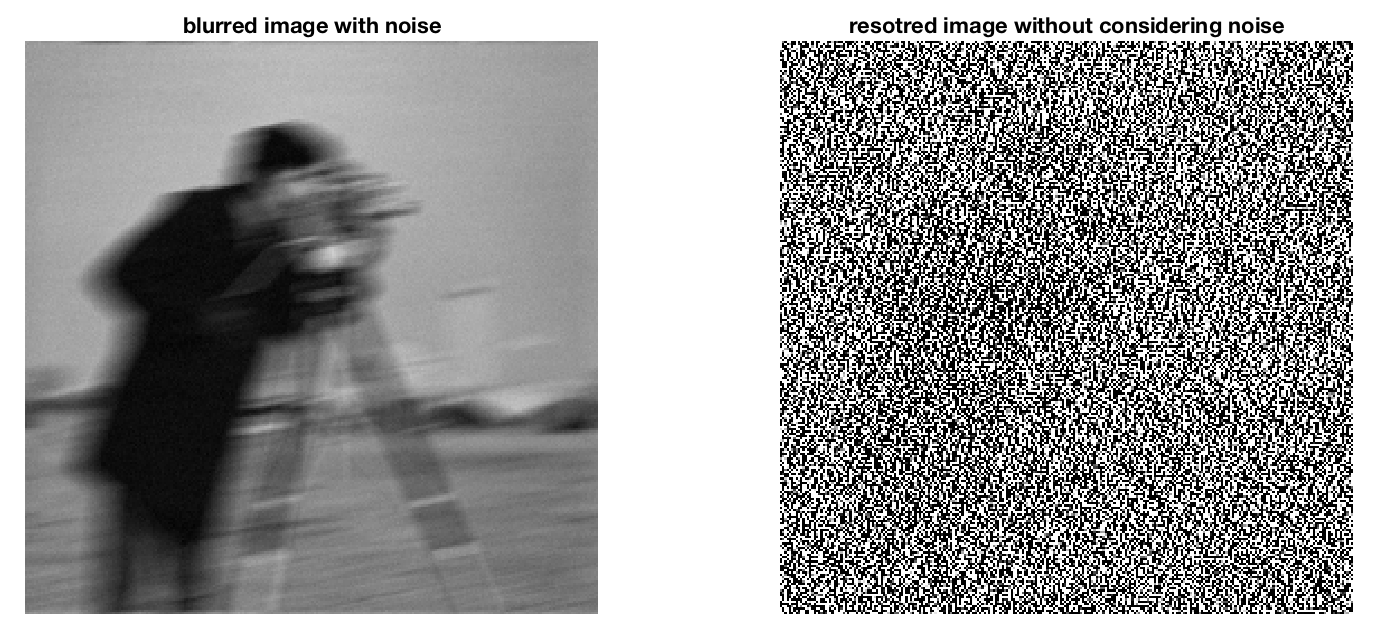### 影象復原的逆濾波方法

g(x,y)=h(x,y)f(x,y)+η(x,y).其中，h(x,y)是退化函式在時域下的表示，運算子表示時域卷積。由卷積定理可知，時域上的卷積等同於頻域上的乘積，所以上式在頻域中的表述如下：
G(u,v)=H(u,v)F(u,v)+N(u,v)F̂ (u,v)=H(u,v)F(u,v)+N(u,v)H(u,v)=F(u,v)+N(u,v)H(u,v)

I = im2double(imread('cameraman.tif'));
LEN = 21; THETA = 11;
PSF = fspecial('motion', LEN, THETA);
blurred = imfilter(I, PSF, 'conv', 'circular');

result1 = mydeconvwnr(blurred, PSF, 0);

subplot(1,3,1),imshow(I),title('original image');
subplot(1,3,2),imshow(blurred),title('blurred image');
subplot(1,3,3),imshow(result1),title('restored image');noise_mean = 0;
noise_var = 0.0001;
blurred_noisy = imnoise(blurred, 'gaussian', noise_mean, noise_var);
estimated_nsr = 0;
result2 = mydeconvwnr(blurred_noisy, PSF, estimated_nsr);
subplot(1,2,1),imshow(blurred_noisy ),title('blurred image with noise');
subplot(1,2,2),imshow(result2),title('resotred image without considering noise');### 在MATLAB中實現維納濾波

F̂ (u,v)=[H(u,v)|H(u,v)|2+PN(u,v)/PS(u,v)]G(u,v)=[1H(u,v)|H(u,v)|2|H(u,v)|2+PN(u,v)/PS(u,v)]G(u,v)